# Korteweg-de Vries equation

Jump to: navigation, search

KdV-equation

The equation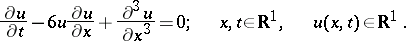It was proposed by D. Korteweg and G. de Vries  to describe wave propagation on the surface of shallow water. It can be interpreted using the inverse-scattering method, which is based on presenting the KdV-equation in the formwhere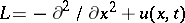is the one-dimensional Schrödinger operator andThe Cauchy problem for the KdV-equation is uniquely solvable in the class of rapidly decreasing functionswith the initial condition: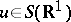(where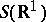is the Schwartz space). Let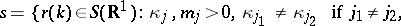be the scattering data for the Schrödinger operator with potential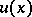,the discrete spectrum,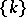the continuous spectrum and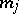normalization coefficients of the eigen functions. Then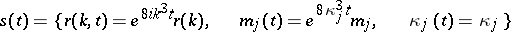are the scattering data for the Schrödinger equation with potential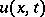, and the solutionis determined from the scattering datausing a certain integral equation. If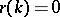, the latter equation can be solved explicitly; the potentials thus obtained are known as reflection-free, and the corresponding solutions of the KdV-equation are known as-solitons (see Soliton).

The KdV-equation may be written in Hamiltonian formhere the phase space isand the Poisson brackets are defined by the bilinear form of the operator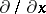. The mapping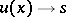is a canonical transformation to variables of action-angle type. In terms of these new variables the Hamilton equations can be integrated explicitly. The KdV-equation possesses infinitely many integrals of motion: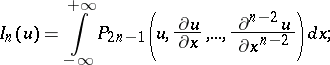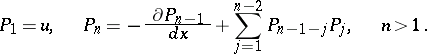All these integrals of motion are integrals in involution, and the Hamiltonian systems that they generate (known as higher Korteweg–de Vries equations) are completely integrable.

Using the integral equations of the inverse problem, one can also find the solution of the Cauchy problem for step-type initial data: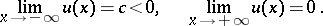As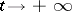in a neighbourhood of the front, the solutiondecomposes into non-interacting solitons — this is the process of step disintegration.

In the case of the Cauchy problem with periodic initial data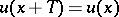,, the analogue of reflection-free potentials is provided by potentials for which the Schrödinger operator has finitely many forbidden zones — finite-gap potentials. Periodic and almost-periodic finite-gap potentials are stationary solutions of the higher KdV-equations; the latter constitute completely-integrable finite-dimensional Hamiltonian systems. Any periodic potential can be approximated by a finite-gap potential. Let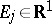,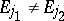if(), be the edges of the bands, andthe hyperelliptic curveover the field. Then real-valued almost-periodic potentials with the above band edges, as well as solutions of the Cauchy problem, are expressible in terms of-functions on the Jacobi variety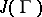of the curve. Subject to certain conditions on the edges, the resulting solutions will be periodic. If one drops the conditions, one obtains complex-valued solutions of the KdV-equation (possibly with poles), which are also called finite-gap potentials.